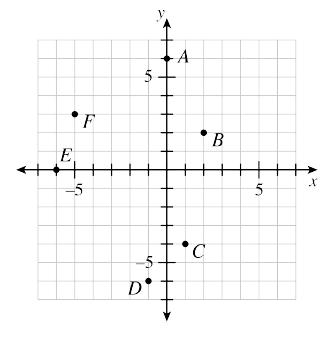### Home > ACC6 > Chapter 6 Unit 6 > Lesson CC1: 6.2.5 > Problem6-119

6-119.This problem is a checkpoint for locating points on a number line and on a coordinate graph. It will be referred to as Checkpoint .

1. Indicate the approximate location of each number on a number line.

$−2$, $4$, $−1.7$, $\frac { 3 } { 4 }$, $−0.2$$-\frac{10}{3}$$4\frac{1}{5}$, $150\%$

2. What are the coordinates of each named point on the coordinate graph?